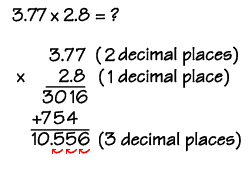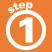Home    |    Teacher    |    Parents    |    Glossary    |    About UsTo multiply decimal numbers:

1. Multiply the numbers just as if they were whole numbers.
• Line up the numbers on the right - do not align the decimal points.
• Starting on the right, multiply each digit in the top number by each digit in the bottom number, just as with whole numbers.Homework Help | Pre-Algebra | NumbersEmail this page to a friendSearch·  Place value·  Decimal numbers·  Estimating and     rounding·  Adding / subtracting     decimals·  Multiplying decimals·  Dividing decimals·  Percent·  Exponents·  Square roots·  Signed integers·  Adding and     subtracting integers·  Multiplying and     dividing integers·  Properties of integersFirst Glance In Depth Examples WorkoutMultiplying decimals